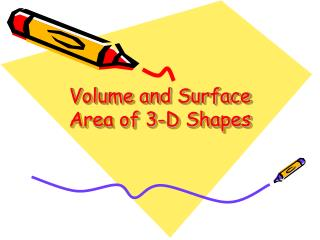DownloadDownload PresentationVolume and Surface Area of 3-D Shapes

# Volume and Surface Area of 3-D Shapes

Télécharger la présentation## Volume and Surface Area of 3-D Shapes

- - - - - - - - - - - - - - - - - - - - - - - - - - - E N D - - - - - - - - - - - - - - - - - - - - - - - - - - -
##### Presentation Transcript

1. Volume and Surface Area of 3-D Shapes

2. VOLUME • Volume is the measure of space inside a 3-D object. • Imagine filling the classroom with boxes measuring 1 cubic foot. How much would we use? • Area of the base x height = volume of a prism or cylinder • (Area of the base x height) ÷ 3 = volume of a pyramid

3. SURFACE AREA • Surface area is the measure of all faces on a 3-D object. • Imagine covering the floor, walls, and ceiling of this room with tiles measuring 1 square foot. How many would we use? • Surface Area = sum of areas of all faces and bases

4. Mary wants to fill the box below with candy hearts and then wrap the box in Valentine’s paper. The box is 6 inches long, 6 inches wide, and 12 inches high. Each pack of candy is 3 cubic inches. How many packs of candy will she need? She has a piece of wrapping paper that is 500 square inches. Will that be enough? Will there be any wrapping paper left over? V = 6 x 6 x 12 = 432 cubic inches 432 ÷ 3 = 144 packs of candy SA = 2(6x6) + 2(6x12) + 2(6x12) = 360 square inches 500 – 360 = 140 square inches left

5. Bill is working on a farm for the summer. He is told by Farmer Brown to remove the top of the silo, fill it with grain, and then paint the outside of the silo to match the barn. The silo is 30 feet tall, and 6 feet across the top. One acre of harvested grain is 64 cubic feet. Bill estimates it will take 10 acres of harvested grain to fill the silo. Is his estimate accurate? Why/why not? One can of red paint will cover 100 square feet. How many cans of red paint will Bill need? V = ∏r2h V = 3.14 x 3 x 3 x 30 Volume = 847.8 cubic feet 64 x 10 = 640 847.8 – 640 = 207.8 cubic feet of space left over. Bill needs about 13 acres of harvested grain to fill the silo. SA = area of 2 circles + area of rectangle. ∏r2= 3.14 x 3 x 3 = 28.26 square feet The rectangle is wrapped around the circle, so its length is the circumference of the circle. C = ∏d = 3.14 x 6 = 18.84 A = lw= 18.84 x 30 = 565.2 square feet SA = 28.26 + 28.26 + 565.2 = 621.72 square feet Bill needs 7 cans of paint, because 6 cans will only cover 600 square feet.

6. A theme park is creating a new statue to honor the recently deceased Mr. Tour I. Angle. You are on the planning committee. The designs available are a triangular prism and a square pyramid. The statues will need to be painted and filled with concrete. The triangular prism would have a 12 foot-long base, stand 8 feet tall, and be 8 feet thick with a slant height of 10 feet. The square pyramid would have an equilateral base of 12 feet, and stand 8 feet tall with a slant height of 10 feet. Each face height would be 8 feet. A \$20 can of paint will cover 100 square feet. A cubic foot of concrete costs \$5. How much will each statue cost? Which is the best choice, if the park is trying to save money? Prism V = (½ bh)h = 48 x 8 =384 cubic feet 384 x\$5 = \$1920 Prism SA = area of 2 triangles + area of 3 rectangles = 48+48+96+80+80 = 352 square feet \$20 x 4 = \$80 Pyramid V = lwh ÷ 3 = 384 cubic feet 384 x\$5 = \$1920 Pyramid SA = area of 4 triangles + area of square base = 48+48+48+48+144 = 336 square feet \$20 x 4 = \$80

7. HOMEWORK ASSIGNMENT • Write your own word problem involving volume and surface area. • You may not use one of the previous examples. • If you bring a visual aid to go with your problem, you will receive extra credit.